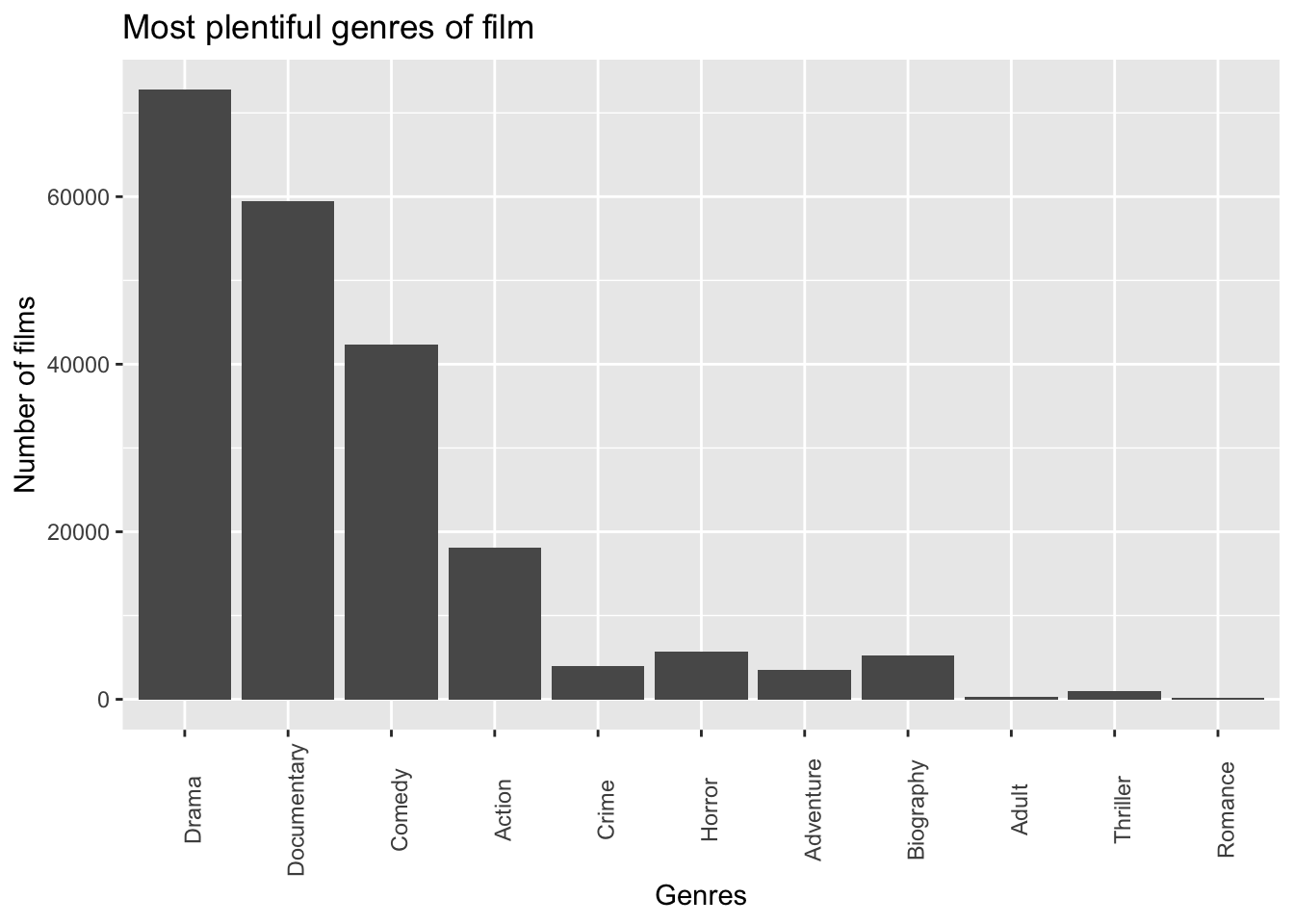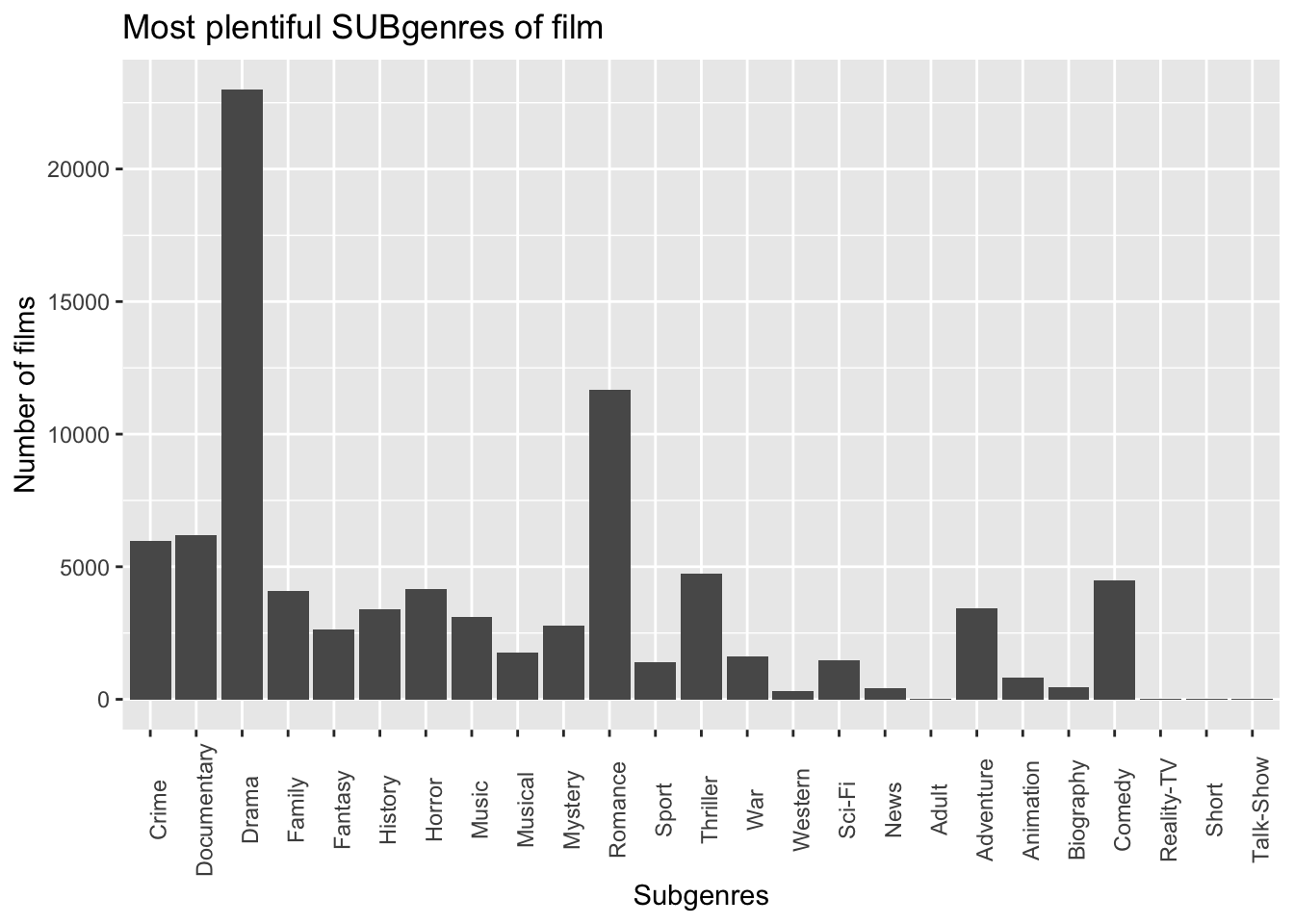This EDA takes a look at data from IMDb to analyze trends in movie and TV genres and subgenres over time. Specifically, I’m using the “title.basics” dataset from IMDb, which was obtained in October of 2020. I have also imported a dataset of the highest grossing films globally, compiled by Aaron O’Neill on Statista. (https://www.statista.com/statistics/1072778/highest-grossing-movie-annually-historical/)

Some core questions I’d like to answer in this EDA:

• What have the most popular (“popular”, in the context of this EDA, meaning plentiful) film genres and subgenres been in the last 60 years? How have the most popular genres changed over the decades?

• Do the highest grossing films globally give us an idea of genre trends? In other words, did films like “Titanic” lead to a resurgence in romance films?

• Similarly, what have the most popular TV genres and subgenres been in the last 60 years? How have these changed over the decades?

To create a nice time window for the data, I’ve limited my analysis to only films and television from 1959 to 2019.

``## ── Attaching packages ─────────────────────────────────────── tidyverse 1.3.0 ──``
``````## ✓ ggplot2 3.3.2     ✓ purrr   0.3.4
## ✓ tibble  3.0.4     ✓ dplyr   1.0.2
## ✓ tidyr   1.1.2     ✓ stringr 1.4.0
## ✓ readr   1.4.0     ✓ forcats 0.5.0``````
``````## ── Conflicts ────────────────────────────────────────── tidyverse_conflicts() ──

## Setting up the movie data

``````#importing the raw IMDb data (filtered)
#importing the raw highest-grossing film data
#importing the filtered IMDb movie data

highest_grossing <- highest_grossing %>%
separate(movie, into = c("year", "movie"), sep = " - ")
#now that the year and movie are separate, removing films that aren't within our time range, along with 1968's Funny Girl (this ranking is only based on U.S. data, which isn't useful for our global study)
highest_grossing <- highest_grossing %>%
filter(year != "****2020") %>%
filter(year != "1968") %>%
filter(year >= 1959) %>%
filter(year <= 2019)
#turning the year value into a double (this'll be useful for graphing the top films later)
highest_grossing <- highest_grossing %>%
mutate(year = as.double(year))

#filtering to our time period
imdb_final_data <- imdb_data %>%
filter(startYear >= 1959) %>%
filter(startYear <= 2019)
#splitting genre and subgenre
imdb_final_data <- imdb_final_data %>%
separate(genres, into = c("genre", "subgenre"), sep = ",")

#creating counts of film by genre and subgenre, by year
imdb_movies <- imdb_movies %>%
group_by(startYear) %>%
mutate(genre_year_count = sum(!is.na(genre))) %>%
mutate(subgenre_year_count = sum(!is.na(subgenre)))

#creating a top_film joined dataset - combining the highest-grossing data with the film details, like genre and subgenre
top_film <- inner_join(highest_grossing, imdb_movies,
c("movie" = "primaryTitle", "year" = "startYear")) %>%
select(-movie, year)

#creating rankings of genre and subgenre based on top_film and the imdb_movies data
genres_over_time <-count(imdb_movies, genre, sort = TRUE) %>%
group_by(startYear)
#fct_lump_lowfreq(genre)
subgenres_over_time <- count(imdb_movies, subgenre, sort = TRUE) %>%
filter(!is.na(subgenre)) %>%
group_by(startYear)

top_films_over_time <- full_join(top_film, genres_over_time,
c("year" = "startYear", "genre" = "genre")) %>%
filter(!is.na(originalTitle))

top_subs_over_time <- full_join(top_film, subgenres_over_time,
c("year" = "startYear", "subgenre" = "subgenre")) %>%
filter(!is.na(originalTitle))

#Making a proportional decade-based genre heatmap
heatmap <- imdb_movies %>% mutate(startYear = as.character(startYear)) %>%
mutate(startYear = fct_collapse(startYear,
"2010s" = c("2019", "2018", "2017", "2016", "2015", "2014", "2013", "2012", "2011", "2010"),
"2000s" = c("2009", "2008", "2007", "2006", "2005", "2004", "2003", "2002", "2001", "2000"),
"1990s" = c("1999", "1998", "1997", "1996", "1995", "1994", "1993", "1992", "1991", "1990"),
"1980s" = c("1989", "1988", "1987", "1986", "1985", "1984", "1983", "1982", "1981", "1980"),
"1970s" = c("1979", "1978", "1977", "1976", "1975", "1974", "1973", "1972", "1971", "1970"),
"1960s" = c("1969", "1968", "1967", "1966", "1965", "1964", "1963", "1962", "1961", "1960")
)) %>%
select(genre, startYear) %>%
filter(startYear != "1959")

heatmap <-count(heatmap, genre, sort = TRUE) %>%
group_by(startYear) %>%
mutate(prop = n / sum(n))``````

## Movie graphs

``````#bar graph of genres from 1959-2019
imdb_movies %>%
filter(rank(genre_count) > 1000) %>%
mutate(genre = fct_reorder(genre, genre_count)) %>%
ggplot() +
geom_bar(aes(x = genre)) +
labs(x = "Genres", y = "Number of films", title = "Most plentiful genres of film ") +
theme(axis.text.x = element_text(angle = 90))````````````#bar graph of subgenres from 1959-2019
imdb_movies %>%
filter(rank(subgenre_count) > 1000) %>%
filter(!is.na(subgenre)) %>%
mutate(subgenre = fct_reorder(subgenre, subgenre_count)) %>%
ggplot() +
geom_bar(aes(x = subgenre)) +
labs(x = "Subgenres", y = "Number of films", title = "Most plentiful SUBgenres of film") +
theme(axis.text.x = element_text(angle = 90))````````````#Let's switch from aggregate graphs to graphing the popularity per year - again by genre and subgenre.

ggplot() +
geom_line(data = genres_over_time, mapping = aes(x = startYear, y = n, color = genre)) +
geom_point(data = top_films_over_time, mapping = aes(x = year, y= n, color = genre)) +
labs(x = "Year", y = "Number of films globally", title = "Trends in film genres globally from 1959-2019")``````